Payal Tandon
Co-founder, e-GMAT
Welcome to e-GMAT Support!
I am Payal, Co-Founder of e-GMAT.
Feel free to ask any Query.We will be contacting you soon on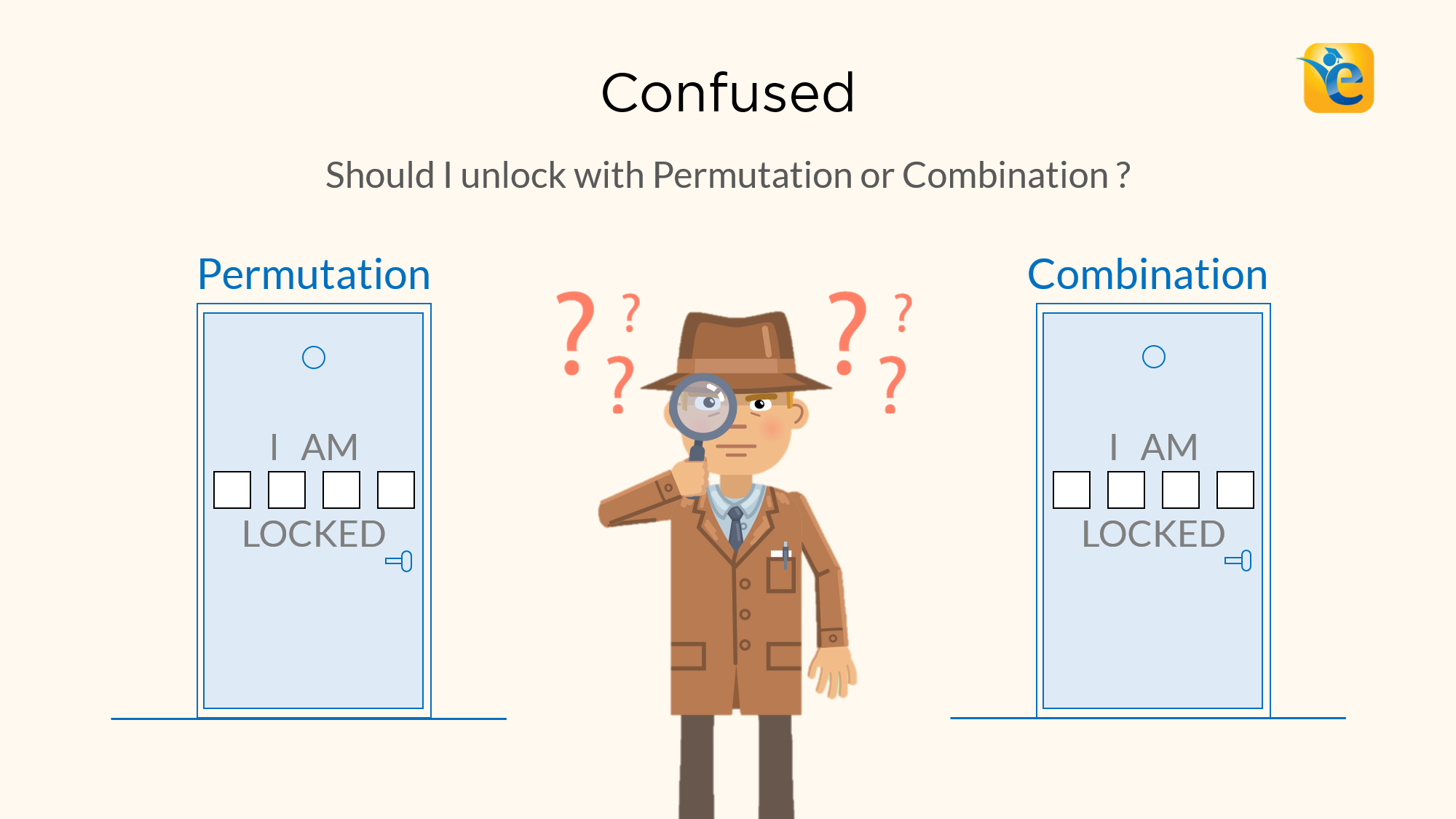# Difference between Permutation and Combination explained with examples

Permutations and Combinations is a topic full of conundrums. The biggest one is, understanding the difference between permutation and combination. Should I solve this...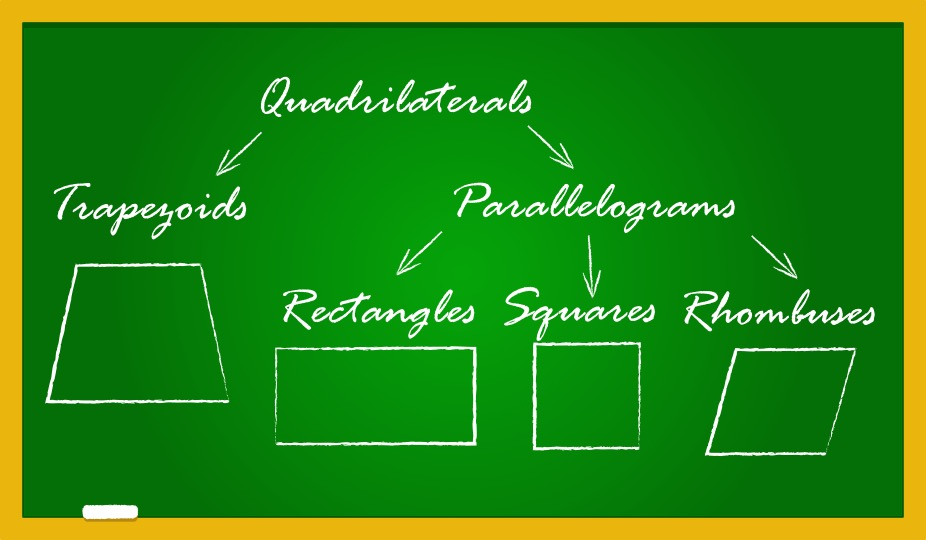# Properties of Quadrilaterals – Rectangle, Square, Parallelogram, Rhombus, Trapezium | 2023

In Euclidean geometry, a quadrilateral is a four-sided 2D figure whose sum of internal angles is 360°. The word quadrilateral is derived from two Latin words ‘quadri’ and...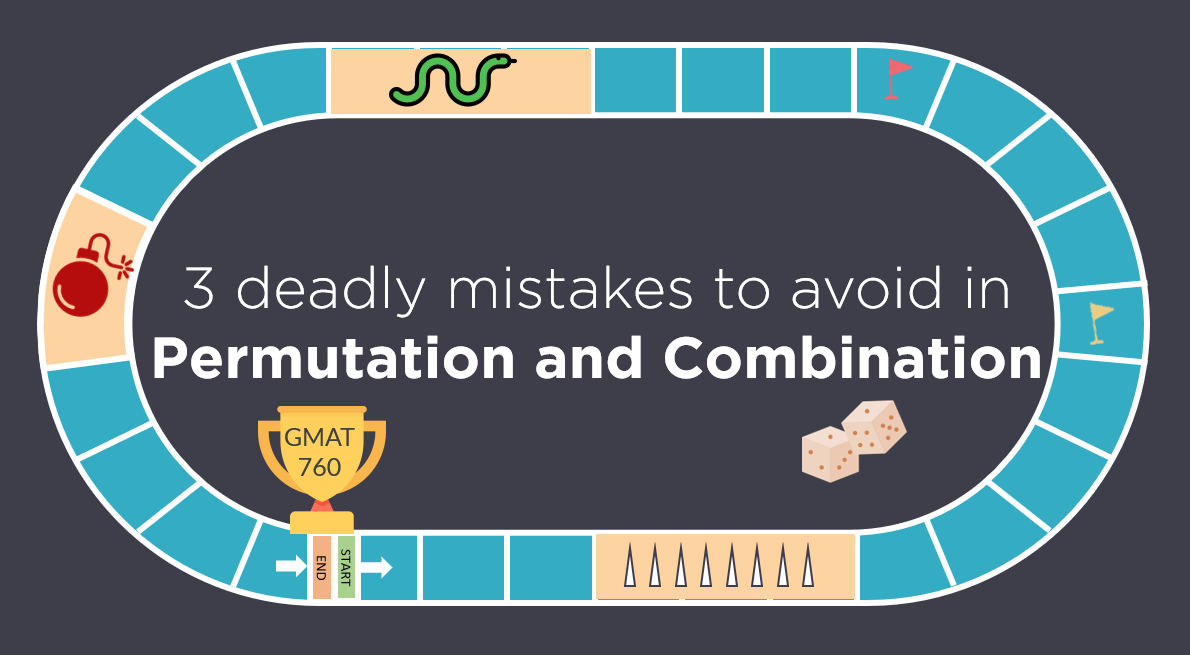# Permutation and Combination | Avoid these 3 Mistakes | GMAT Quant

Like any GMAT Quant topic, Permutation and Combination has its own traps. Most students fall in these traps and ultimately, are not able to secure their target GMAT score....# Properties of Quadrilaterals – Rectangle, Square, Parallelogram, Rhombus, Trapezium | 2023

In Euclidean geometry, a quadrilateral is a four-sided 2D figure whose sum of internal angles is 360°. The word quadrilateral is derived from two...# Circle Formulas -What is a Circle and its properties? (Definition & Examples)

A circle is a closed shape formed by tracing a point that moves in a plane such that its distance from a given point is constant. The word circle is...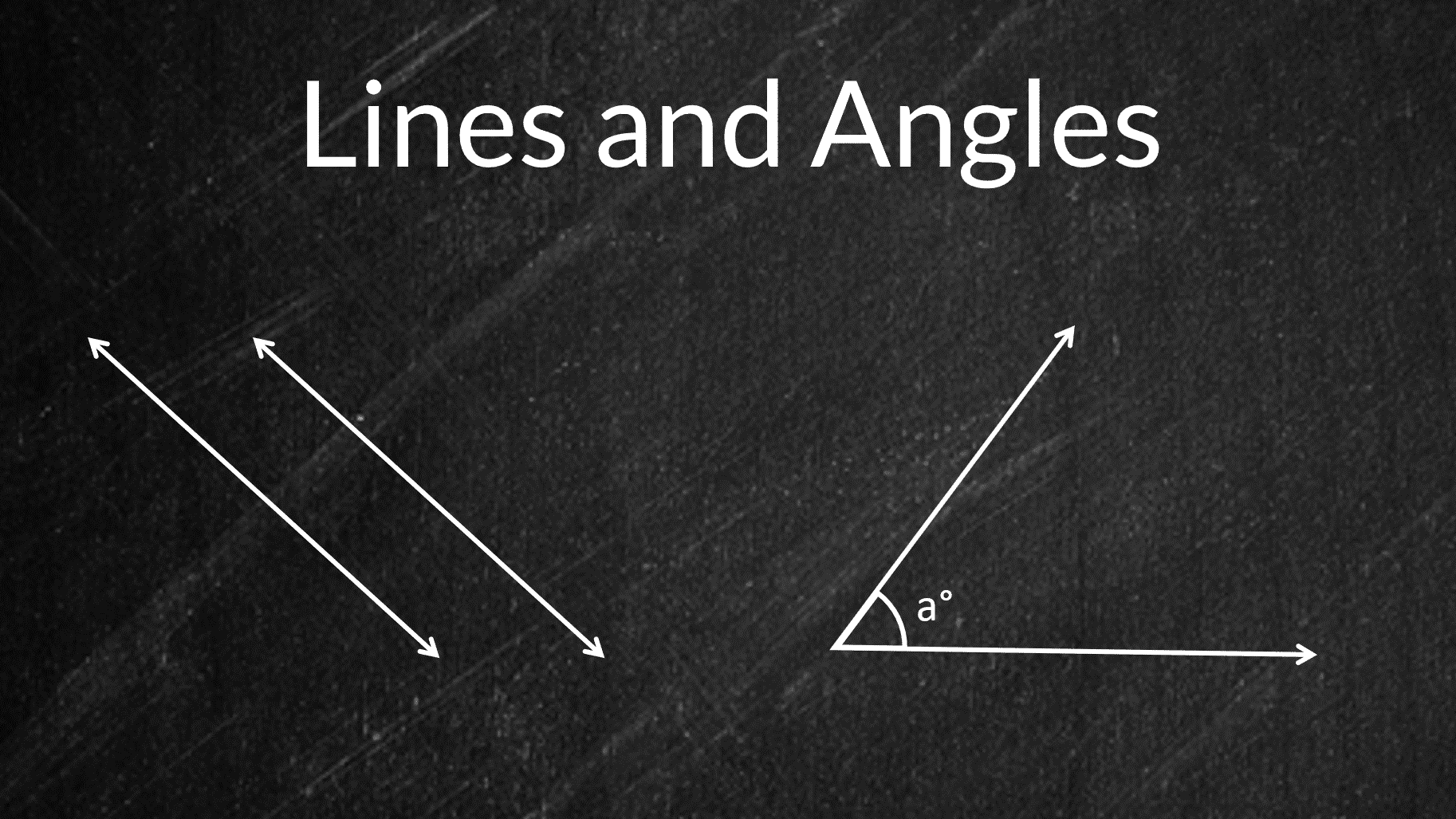# Lines and Angles – Definitions, Properties, Types, Practice Questions

Ancient mathematicians introduced the concept of lines to represent straight objects which had negligible width and depth. Considered as a breadth...# Improve accuracy in GMAT Math questions on Polygons

A polygon is a two-dimensional closed figure made up of at least 3 line segments. Polygons, as a topic, will pose some of the most challenging GMAT...# Properties of Triangle – types & formulas [Video & Practice]

In this article, we are going to learn about the simplest form of a polygon, a triangle. All polygons can be divided into triangles, or in other...

# More articles

## Properties of Quadrilaterals – Rectangle, Square, Parallelogram, Rhombus, Trapezium | 2023

A 9 min read In Euclidean geometry, a quadrilateral is a four-sided 2D figure whose sum of...

## Circle Formulas -What is a Circle and its properties? (Definition & Examples)

A 8 min read A circle is a closed shape formed by tracing a point that moves in a plane such that...

## Lines and Angles – Definitions, Properties, Types, Practice Questions

A 8 min read Ancient mathematicians introduced the concept of lines to represent straight objects...

## Improve accuracy in GMAT Math questions on Polygons

A 10 min read A polygon is a two-dimensional closed figure made up of at least 3 line segments....

## The dimensions of a ream of paper are 8 1/2 inches by 11 inches by 2 1/2 inches. The inside dimensions of a carton that will hold exactly 12 reams of paper could be – OGQR 2020 Question #93 with Solution

A 1 min read OGQR 2020: Question No. 93 The dimensions of a ream of paper are 8 1/2 inches by 11...

## In the figure above, is the area of triangular region ADE equal to the area of rectangular region ABCD? – OGQR 2020 Question #206 with Solution

A 2 min read OGQR 2020: Question No.206 In the figure above, is the area of the triangular region ADE equal to the area of rectangular region ABCD? x = 10 and y = 5.x = 2y SourceOGQR 2020TypeData...

## The figure above is constructed by separating a circular region into 6 equal parts and rearranging the parts as shown. If the diameter of the circle is d, what is the perimeter of the figure above? – OGQR 2020 Question #89 with Solution

A 2 min read The figure above is constructed by separating a circular region into 6 equal parts and rearranging the parts as shown. If the diameter of the circle is d, what is the perimeter of the figure above? Source OGQR 2020...

## In a rectangular coordinate system, straight-line k passes through points (0, 0) and (3, 2). Which of the following are coordinates of a point on k? – OGQR 2020 Question #78 with Solution

A 2 min read OGQR 2020: Question No. 78 In a rectangular coordinate system, straight-line k passes through points (0, 0) and (3, 2). Which of the following are coordinates of a point on k? SourceOGQR 2020TypeProblem...

## In the figure above, the vertices of ΔOPQ and ΔQRS have coordinates as indicated. Do ΔOPQ and ΔQRS have equal areas? – OG 2020 Question #325 with Solution

A 2 min read OG 2020: Question No. 325 In the figure above, the vertices of ΔOPQ and ΔQRS have coordinates as indicated. Do ΔOPQ and ΔQRS have equal areas? b = 2ad = 2c SourceOG 2020TypeData...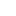###### Don't limit yourself!# Achieve 740+on the GMAT in 30 days!Sign up for our free trial and get:400+ Practice questions with detailed solutions10+ hours of AI-driven video lessonsAdaptive mock test with ESR+ analysis